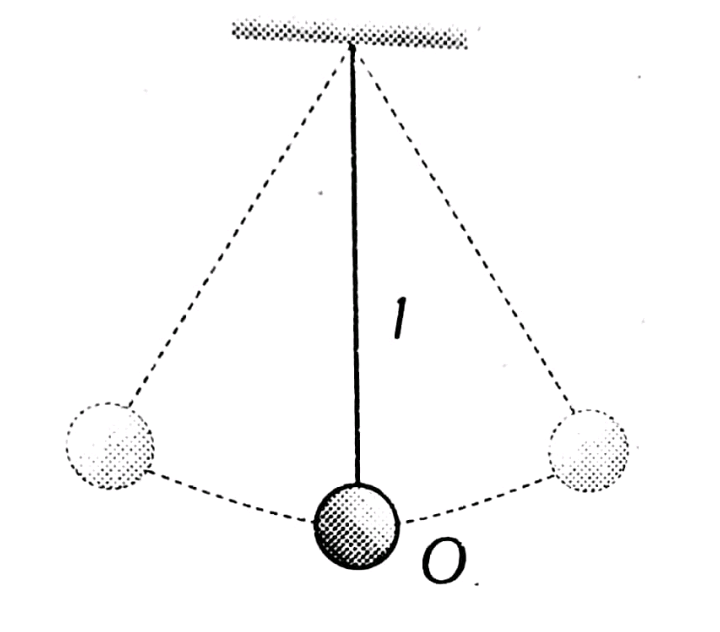Q

# Answer please! The length of simple pendulum is increased by 1% its time period will

The length of simple pendulum is increased by 1% its time period will

• Option 1)

Increase by 1%

• Option 2)

Increase by 0.5%

• Option 3)

Decrease by 0.5%

• Option 4)

Increase by 2%

107 Views

As we have learnt,

Simple Pendulum -

.

- whereinPercentage increment in  (percentage increment in l) = 0.5%.

Option 1)

Increase by 1%

Option 2)

Increase by 0.5%

Option 3)

Decrease by 0.5%

Option 4)

Increase by 2%

Exams
Articles
Questions